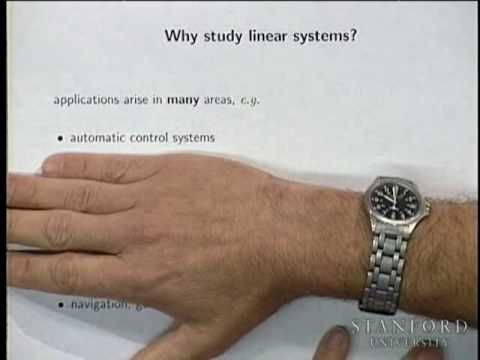# Stanford► Play all

# Lecture Collection | Linear Dynamical Systems

• Stanford
• 20 videos
• 133,817 views
• Last updated on Sep 25, 2014
Introduction to Linear Dynamical Systems (EE263) is the introduction to applied linear algebra and linear dynamical systems, with applications to circuits, signal processing, communications, and control systems. Topics include: Least-squares aproximations of over-determined equations and least-norm solutions of underdetermined equations. Symmetric matrices, matrix norm and singular value decomposition. Eigenvalues, left and right eigenvectors, and dynamical interpretation. Matrix exponential, stability, and asymptotic behavior. Multi-input multi-output systems, impulse and step matrices; convolution and transfer matrix descriptions. Control, reachability, state transfer, and least-norm inputs. Observability and least-squares state estimation. EE263 covers some of the same topics, but is complementary to, CME200.
Introduction to Linear Dynamical Systems (EE263) is the introduction to applied linear algebra and linear dynamical systems, with applications to circuits, signal processing, communications, and control systems. Topics include: Least-squares aprox...